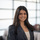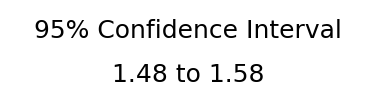# Confidence Interval Printout - PythonLet's say we want a printout of our confidence interval for an entire sample (Note, if you're looking for a visual of a confidence interval over time, check out the post here!) The solution here requires Periscope Data's Python/R Integration as we'll be using Python's Scipy package and matplotlib text charts.

Our SQL output is a dataframe (df) with one column of a list of values.  Notice that the function created in the Python snippet also takes in the following optional parameters for added customization:

• interval: the width of the Confidence Interval. Default is set to 0.95 (95%)
• method: whether we use the t distribution or a z/normal distribution to calculate the confidence interval. Default is set to a 't' distribution. We recommend verifying that your data is normally distributed before using the z distribution statistic.

The snippet is written in Python 3.6

```# SQL output is imported as a pandas dataframe variable called "df"
import pandas as pd
from scipy import stats
import math
import matplotlib.pyplot as plt
import numpy as np

#Function: CI_prinout, a function that outputs a number overlay expressing a sample's Confidence Interval
#Inputs: a dataframe with one column of values. Optional paramater interval for the size of the confidence interval (default is 0.95). Option parameter method that specifies whether the confidence interval will be calculating using the t distribution or a z/normal distribution.
#Outputs: a matplotlib text chart with the % confidence interval and the lower and upper bounds
def CI_printout(series, interval = 0.95, method = 't'):
mean_val = series.mean()
n = series.count()
stdev = series.std()
if method == 't':
test_stat = stats.t.ppf((interval + 1)/2, n)
elif method == 'z':
test_stat = stats.norm.ppf((interval + 1)/2)
lower_bound = mean_val - test_stat * stdev / math.sqrt(n)
upper_bound = mean_val + test_stat * stdev / math.sqrt(n)

fig = plt.figure()
plt.axis('off')
plt.gcf().set_size_inches(8, 2)
plt.xticks([])
plt.yticks([])

plt.text(.5, .75, str(round(interval * 100))+ '% Confidence Interval', fontsize=25, color='black', ha='center')
plt.text(.5, .35, str(round(lower_bound,2)) + ' to ' + str(round(upper_bound,2)), fontsize=25, color='black', ha='center')

return plt

# Use Periscope to visualize a dataframe or an image by passing data to periscope.output()
periscope.output(CI_printout(df))
```

For the R equivalent of this post, check out the community page here!

Found this useful? Let us know in the comments!